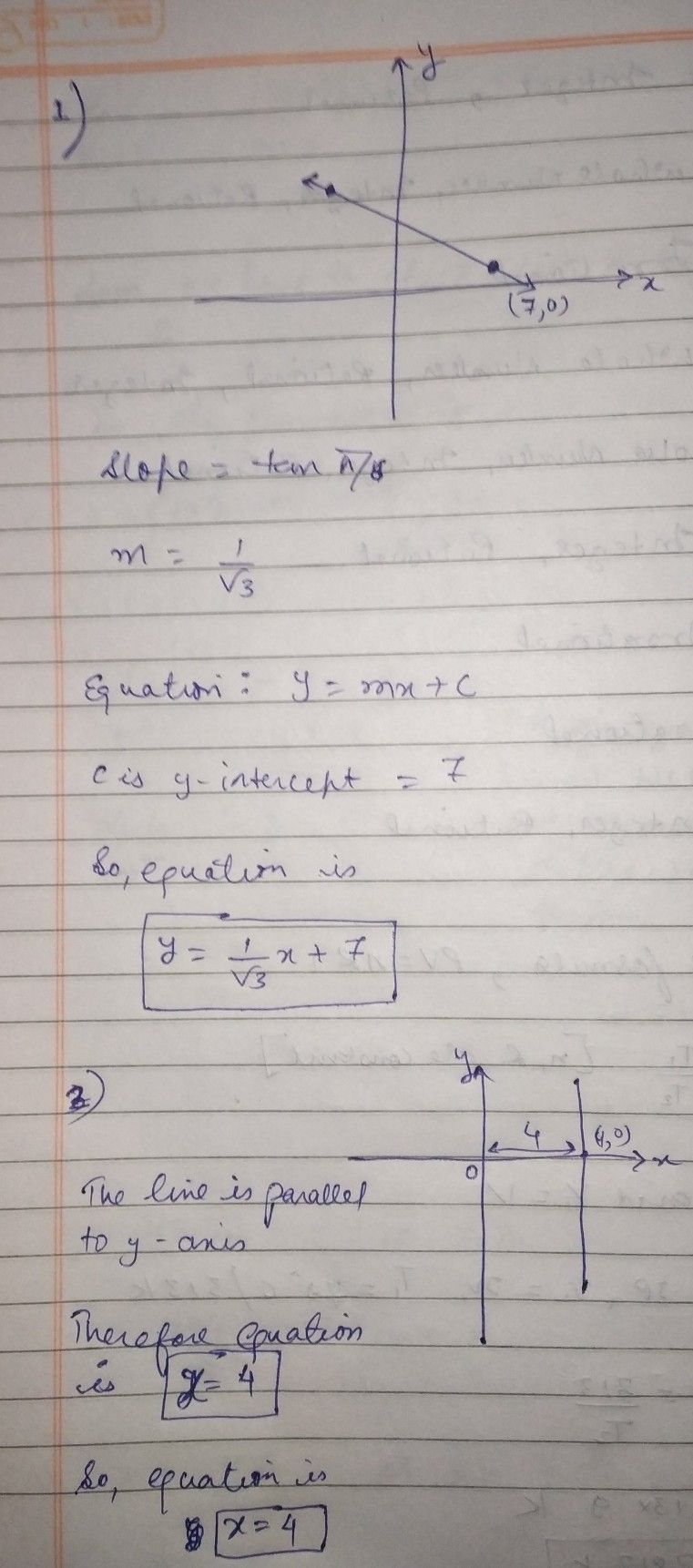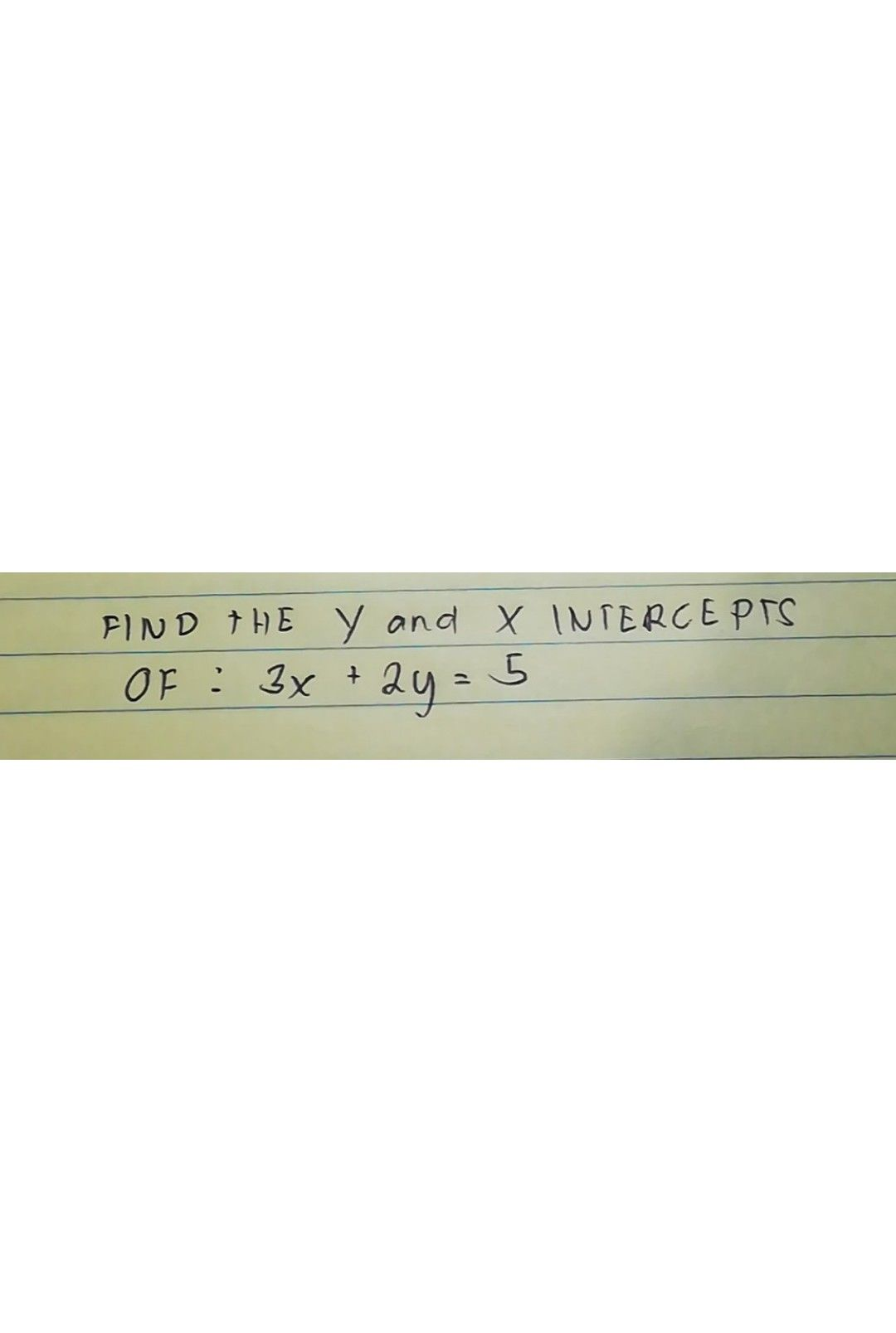Symbol
ProblemLearning Task $4:$ Do the following in your notebook. $A.$ Find the equation of the line given the graph. $1$ $2$ $3$ $4$ $2$ $-$ $=$ $k$ $k$ $k$
7th-9th grade
Other
SolutionQanda teacher - mamtaStudentHow???Qanda teacher - mamta
sorry dear. please ask one question at a time
please ask seperatelyStudent
OkayQanda teacher - mamta
i m really sorry
but this is the policy of this appStudent
Okay i understand :((Qanda teacher - mamta
??